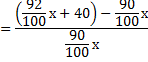# Profit and Loss for IBPS PO : Set 2

Q.1) If the percentage profit when it is calculated on its cost price is 25%, then what is the percentage profit when it is calculated on selling price?

a) 25%

b) 30%

c) 20%

d) 15%

e) None of these

c

Let cost price be Rs.100

Profit=25

Selling price=125/100×100=125

Required percenatge=25/125×100=20%

Q.2) A book seller sells two books for Rs.500 each. If he sold one of the books at 15% profit, then what is the loss percentage of another book to make neither profit nor loss in total?

a) 15%

b) 20%

c) 5%

d) 10%

e)12%

a

Cost price of two books=500+500=1000

Selling price of two books=115×500/100+x=1000

x=425

Required percentage=75/500×100=15%

Q.3) Rajiv buys a land for Rs.21 lakhs. He sells  3/7th of the land at a loss of 8%. now He wants to make a profit of 15% on the whole transaction by selling the remaining land. At what percentage profit should he sell his remaining land?

a) 28.85%

b) 30.05%

c) 25.55%

d) 32.25%

e) None of these

d

Cost price of land=Rs.21 lakh

Selling price=21× 115/100=24.15 lakh

3/7×21×92/100+x=24.15

x=24.15-8.28=15.87

cost price of remaining land=21-3/7×21=21-9=12

% profit=(15.87-12)/12×100=32.25%

Q.4) While selling a belt a shopkeeper gives a discount of 11%. If he gives a discount of 14% he earns Rs.120 less as profit. What is the marked price of the belt?

a) Rs.400

b) Rs.4500

c) Rs.4000

d) Rs.2800

e) None of these

c

Let marked price of belt =x

89/100 x-86/100 x=120

3x =12000

x=4000

Q.5) The cost price of 10 articles is the same as the selling price of x articles. If the profit is 25%, then what is the value of x?

a) 7

b) 8

c) 11

d) 12

e) 10

b

Let C.P. of each article be Re. 1

C.P. of x articles = Rs. x.

S.P. of x articles = Rs. 10.

Profit = Rs. (10 – x).

25/100=(10-x)/x

1000 – 100x = 25x

125x=1000

x=8

Alternate method

If cost price of x articles is same as selling price of y articles, then

Profit percentage=(x-y)/y×100

25= (10-x)/x×100

X=8

Q.6) The percentage profit earned by selling an article for Rs. 2840 is equal to the percentage loss incurred by selling the same article for Rs.2220. At what price should the article be sold to make 5% loss?

a) Rs.2115.25

b) Rs.2510.50

c) Rs.2403.50

d) Rs.2415.50

e) None of these

c

Cost price=(2840+2220)/2=2530

Required selling price=2530×95/100=Rs.2403.50

Q.7) If watches bought at prices ranging from Rs.4000 to Rs.5500 are sold at prices ranging from Rs.5000 to Rs.6500, what is the greatest possible profit that might be made in selling 10 watches?

a) Rs.12000

b) Rs.14000

c) Rs.13500

d) Rs.25000

e) Rs.15500

d

Least Cost Price = Rs. (4000 ×10) = Rs.40000.

Greatest Selling Price = Rs. (6500 × 10) = Rs.65000

Required profit = 65000-40000=25000

Q.8) Divya got 20% discount on the labelled price of an article and sold it for Rs. 550 with 10% profit on the price she bought. What was the labelled price?

a) Rs 650

b) Rs 725

c) Rs 625

d) Rs 650

e) None of these

c

Selling price=550/110×100=500

Label price=500/80×100=625

Q.9) Due to reduction of 15% in price of badam a customer can purchase 3 kg badam more for Rs.200. what is original price of 1 kg badam?

a) Rs.12.24

b) Rs.11.76

c) Rs.11.56

d) Rs.10.64

e) None of these

b

Cost of 3 kg badam after 15% price reduction=15/100×200=30

cost price of 1 kg badam=30/3=Rs.10

Q.10) A man bought an umbrella and sold it at a loss of 8%. If he had bought it at 10% less and sold it for Rs.40 more, he would have made a profit of 11.11%. At what price the man bought an umbrella?

a) Rs 420

b) Rs 450

c) Rs 500

d) Rs 550

e) None of these

c

Let cost price of the umbrella be x.

Selling price=92/100 x

If C.P=90/100 x10x=92x+4000-90x

8x=4000

x=500